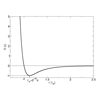#### You may also like### Integration Matcher

Can you match the charts of these functions to the charts of their integrals?### Brimful

Can you find the volumes of the mathematical vessels?### Lennard Jones Potential

Investigate why the Lennard-Jones potential gives a good approximate explanation for the behaviour of atoms at close ranges

# Interpolating Polynomials

##### Age 16 to 18 Challenge Level:

Often you'll be given a polynomial and are asked to find what its value is at some values of $x$. In this problem, we'll ask you to go the other way around: we give you a set of co-ordinates with distinct $x$ values, and you try to find a polynomial that goes through all of them.

First you will prove that such a polynomial exists, then you'll prove some results about the uniqueness of that polynomial.

### Existence

First, draw some points on a graph to convince yourself of the following:

• for any two points, you can draw a straight line between them.
• for three points, you can't always fit them on a straight line, but you might be able to fit them to a quadratic.
• you can draw four points that a quadratic couldn't possibly fit, but maybe a cubic would do.

Now for the algebra: if I give you two points $(x_1,y_1)$ and $(x_2,y_2)$ with $x_1\not=x_2$, you should be able to find the equation of a straight line going through both. The standard way of doing this is to state that the equation of a straight line is $y=mx+c$, calculate $m$ via the formula $m=\frac{y_2-y_1}{x_2-x_1}$ then calculate $c$ via $c=y_1-mx_1$ and you're done. (Why is it important that $x_1\not=x_2$?)

I don't like this method, because it doesn't give me any clues as to how I might fit a curve to three points, or four points, or anything else. Here's an alternative way of thinking about the problem:

1. Find an equation that will fit the first point. The most obvious such would be $y=y_1$.

2. Now, find out by how much you miss the second point, say $d=y_2-y_1$.

3. How can you modify your existing equation so that it hits the second point, without ruining the fact it goes through the first point? Well, if you add any equation to yours which is zero at $x_1$, then the result will have the same value at $x_1$, but may be different elsewhere.

4. Straight lines that are zero at $x_1$ are of the form $m(x-x_1)$. What value of $m$ do we need? Well, take $m=1$ and see what happens at $x_2$: our graph has moved by $D=x_2-x_1$. Clearly if we take $m=2$ it'll move by $2D$, or $m=\frac{1}{2}$ would move it by $\frac{1}{2}D$. We want to move it by $d$, so we take $m=\frac{d}{D}$. We then have $y=y_1+\frac{d}{D}(x-x_1)$, which we can expand and simplify if we so wish, and we're done.

Can you generalise this approach to find a quadratic that goes through (1,0), (4,6), and (2,-2)? Can you find a cubic that goes through these points and (0,-2)?

Can you find a general procedure for fitting $n$ co-ordinates? What kind of graph will you need to use?

### Uniqueness

Draw some graphs of some quadratics to convince yourself that if a quadratic is zero in three places, it must be zero everywhere (equivalently, no quadratic crosses the $x$-axis three times). Then, using the Factor Theorem or otherwise, prove it. (Here by quadratic we just mean $y=ax^2+bx+c$ where any of $a$, $b$ or $c$ may be zero).

Hence prove that if two quadratic curves are equal in three places, they must be equal everywhere. So the quadratic you found that went through (1,0), (4,6), and (2,-2) is the only quadratic that does so.

Generalise your results to polynomials of any degree.